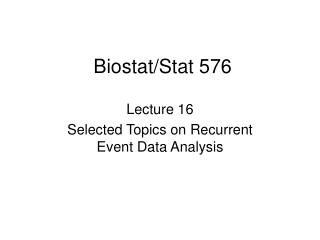Download PresentationBiostat/Stat 576

# Biostat/Stat 576

Download Presentation## Biostat/Stat 576

- - - - - - - - - - - - - - - - - - - - - - - - - - - E N D - - - - - - - - - - - - - - - - - - - - - - - - - - -
##### Presentation Transcript

1. Biostat/Stat 576 Lecture 16 Selected Topics on Recurrent Event Data Analysis

2. Regression Analysis • Regression analysis of gap times • Challenges: last censored observations will complicate any analysis due to biased sampling embedded • One approach is to simply focus on complete gap times • Complete gap times are all right-truncated • Regression methods for right-truncated time-to-event can be adapted

3. Comparability of complete recurrence times

4. Comparable complete gap times of • For , the observation interval is • should satisfy • Similarly, • References • Bhattacharya, et al (1983, Ann. Stat.) • Efron and Petrosian (1999, JASA)

5. Comparability of • Total censoring time • For , • observation time should satisfy • Similarly for ,

6. Conditional on • Total censoring time • Observation time area • Joint density of is proportional to • Due to this comparability, we would have

7. Can we take advantage of this probability structure of comparable complete gap times? • Assume accelerated failure time model • : is subject-specific intercept • : is mean-zero random error of same distributions

8. Random errors • For jth and kth recurrences • Observation interval for random errors

9. Comparability conditions: • Symmetrically • Therefore

10. This motivates estimating equations • Satisfying • Reference: • Wang and Chen (2000, Bmcs)

11. Complete gap times • Right-truncated • Regression analysis for right-truncated failure times • proportional reverse-time hazards models • References: • Lagakos, et al. (1988, Bmka) • Kalbfleisch and Lawless (1991, JASA)

12. Reverse-time hazard function • Proportional reverse-time hazards model • Challenges • How do we construct proper risk sets for right-truncated outcomes? • How do we construct proper risk sets for complete gap times?

13. For usual right-truncated outcomes • Risk set for • Interpretation • Those in the risk set should have fair chance to fail at • Probability of given the risk set

14. For right-truncated complete gap times, • Usual risk sets may not be proper • Observation intervals for those in the risk sets may not be comparable • Right-truncated gap times may become seemingly smaller and smaller just because they occur later in the process • Risk sets need to be adjusted so that those in the risk set should have fair chance to fail not just because they occur later

15. How do we adjust risk sets? • A comprehensive approach • At • This approach is expected to be cumbersome

16. A reduced risk set approach • To include only two gap times in the risk set at a time • Two scenarios: • Include a later gap time in risk set • Later gap time tends to be smaller. So as long as the observation interval is large enough for this gap time to fail at the risk set time, it can be included • Include an earlier gap time in risk set • Earlier gap time tends to be longer. So their advantage in observation time should be offset by early advantage

17. To include a later gap time

18. To include an earlier gap time

19. Conditional on the reduced risk set, the conditional likelihood contribution under the proportional reverse-time model becomes • This is similar to the conditional likelihood in twin studies • Reference • Chen, et al. (2004, Biostat.)

20. Other approaches • Conditional approaches • Marginal approaches • Joint modeling